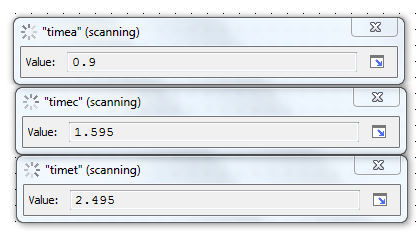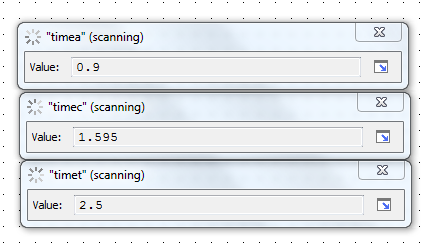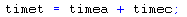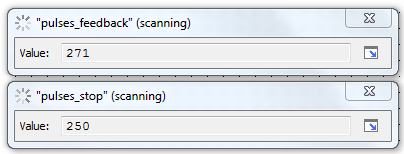# Variable Rounding Issue

I’m totally stumped here.

A little background info: I am using a SNAP-SCM-ST2 module to control a stepper motor. My goal is to be able to control the position of the motor. Since I know the position I want (# of pulses) and speed that I want (freq, delta freq, time slice), I have calculated the time that the motor needs to run in order to reach the desired position and set a downtimer to that time. I have 3 time variables, timea which is the time for the motor to accelerate to its max frequency, timec, the amount of time it runs at the max frequency, and timet, which is simply timea + timec.

Here’s the issue. When the motor is spinning counter-clockwise, timea and timec add together correctly. However, when the motor is spinning clockwise, the result of timea + timec gets rounded to 1 decimal place (see attached images). The inconvenient result of this is that the motor position ends up being just a little bit off of where it should be. What I can’t grasp is how the direction of rotation of the motor has any bearing on the result. Both the equation (timea + timec = timet) and the values of timea and timec are exactly the same either way. Any thoughts?Hmm. Interesting. Could you use the “Move 32 Bits” command for each of those values you show above, and see what the hex values are for each? I’m wondering if the 32 Bits for either the 0.9 or the 1.595 is not EXACTLY the same in each case…

Here’s a little more on floating point math, in case you’re interested:
http://www.opto22.com/site/documents/doc_drilldown.aspx?aid=3472

1 Like

Mary, I was thinking the same thing, but .005 is a large difference. Here is what I come up with:

0x3F66 6667 (Highest value that will display 0.9)
0x3FCC 28F9 (Highest value that will display 1.595)
Result of adding these is still 2.495 displayed (0x401FAE16)

@optonovice Can you post the code where you are adding those two numbers? Is it possible they are getting written to in more than one place?

Also, FWIW, I don’t think you are going to get very precise positioning using timers if 5ms is critical. Do you have any other forms of feedback, like an encoder? Can you describe what you are trying to accomplish?

Idea: If you need to know the exact rotations of your stepper, you could feedback the output of the SNAP-SCM-ST2 into an SNAP-IDC-FAST module if you are using an R1 or EB1 brain (25kHz max) to get an accurate pulse count. You will probably need to decelerate the stepper when you approach your target to precisely stop it.

1 Like

Okay, I found the issue. In the block of code where I calculated the timet to run the motor, the parentheses of an absolute value function were encompassing too much stuff.

Original code:

Fixed:

Fixing that solved the issue. Although I’m not quite sure why…

For reference, freq is the max freq of the motor, freqinit, the initial frequency and I have the if statement because I have specified different motor speeds depending on how far it is going to move. For short moves, it runs at a constant speed, whereas for longer moves, it accelerates up to a faster speed.

FYI - especially when doing math with floats in OptoScript, best to make all your literals floats. E.g. I see a 5 and a 200 in there I’d recommend making 5.0 and 200.0.

I’d also recommend using a naming convention (e.g. pre-pend and “f”) to indicate the data type of your tags.

Because… OptoScript may not handle your data types how you hope/assume it will, so making sure that ALL values are floats will help prevent any piece of your calculation getting converted to a int32 inadvertently, causing weird rounding errors that are tricky to find.

When freq = -400:
`AbsoluteValue(freq <= 200)` ==> `AbsoluteValue(-1)` ==> `1 (true)`
When freq = 0:
`AbsoluteValue(freq <= 200)` ==> `AbsoluteValue(-1)` ==> `1 (true)`
When freq = 400:
`AbsoluteValue(freq <= 200)` ==> `AbsoluteValue(0)` ==> `0 (false)`

Anything non-zero in an if statement is evaluated as true. So whenever freq is <= 200, the expression is evaluating to true. So the AbsoluteValue command was not changing the logic at all.

By performing the AbsoluteValue on just the freq, now you get:

When freq = -400:
`AbsoluteValue(freq) <= 200` ==> `400 <= 200` ==> `0 (false)`
When freq = 0:
`AbsoluteValue(freq) <= 200` ==> `0 <= 200` ==> `-1 (true)`
When freq = 400:
`AbsoluteValue(freq) <= 200` ==> `400 <= 200` ==> `0 (false)`

I hope that was clear enough.

I do this a lot as it is a habit I developed a long time ago. I’m starting to come around though… Mostly because I am spending too much time searching for variables in large projects that pertain to the same piece of equipment.

I’m now starting to use @Beno’s approach:

By prefixing with the equipment name, it speeds up locating the variables. If we had a way of creating folders or grouping variables (structs?) then this wouldn’t be an issue for me. I still do the hungarian thing for looping and temporary variables though.

Thanks guys. I’ll try to do a better job of naming my variables going forward.

Philip, I took your advice and added in a SNAP-IDC5-FAST module to count the pulses. I found that, even with the time rounding issue fixed, the motor was not stopping at the correct number of pulses, so I altered the code to stop the motor when the pulses feedback variable (pulses_feedback) equals or exceeds the number of pulses required to make the move (pulses_stop).

However as you can see, the motor still overshoots its position by several pulses at even low, constant speeds (say, 100hz).You mentioned that the motor may need to be decelerated to precisely stop. Do I simply need to add in a more gradual deceleration rate, or is some kind of feedback control necessary?

Yes, you will probably need to drop below 100Hz. You will need to experiment a little bit - there is a bit of latency for when you decide to stop the stepper and when the command gets to the ST2 module.

These are the delays I can think of:

Your strategy code execution time (probably small)
The strategy/brain loop time (will vary, can be as low as 4ms to 100ms+ depending on how loaded it is)
The TCP stack sending the command to the ST2
The brain converting the TCP command to the ARCnet bus
The ARCnet latency
The latency in the ST2 module

So you are showing 21 steps of overshoot at 100Hz so there was around 210ms of latency, you may need to coast down to just a couple Hz.

Edit: math is hard.

``````if(Direction == Forward) then
LowSpeed=4;
MedSpeed=20;
NormalSpeed=100;
else
LowSpeed=-4;
MedSpeed=-20;
NormalSpeed=-100;
endif

if(GettingClose() and (Speed!=MedSpeed or Speed==0)) then
//Slow down a bit
SetSpeed(MedSpeed);
elseif(GettingRealClose() and (Speed!=LowSpeed or Speed==0)) then
//Run slow
SetSpeed(LowSpeed);
elseif (AreWeThereYet())
//Stop
SetSpeed(0);
else
//Run
SetSpeed(NormalSpeed);
endif``````